# C++ Map: The Complete Step by Step Guide

Maps are part of the C++ STL (Standard Template Library). Maps are part of the C++ STL (Standard Template Library). Maps are typically implemented as Binary Search Tree.

## C++ Map

C++ Map is the dictionary-type associative container, also known as holder objects in the C++ STL. The Maps, as the name suggests storing the values in a mapped fashion, i.e., key-value and a mapped value.

However, while you can have the same mapped values, no two key values can be the same in a single map container. So, let’s see C++ Map in detail.

This is attributed to the fact that the C++ map container uses the key values to store, identify, and sort the values in a map.

Also, internally, the elements in a map are sorted weakly depending upon the internal comparison tool. C++ Map containers support bidirectional iterators.

The bracket operator can easily search the mapped values using their key values.

```mapcontainer_name[key_name];
```

Further, they can also assign mapped values to the key values using the assignment operator.

```mapcontainer_name[ key name ] = mapped value;
```

The primary and most crucial usage of map containers is in the binary search trees.

```std::map <key_datatype, mapped_datatype>
```

See the following example.

```std::map <int, char>;
```

This declares a new map container with the key datatype as integer and the mapped values as characters.

## Program to show usage of Map container in C++.

See the following code.

```#include <iostream>
#include <iterator>
#include <map>
using namespace std;
int main() {
map<int, char> map1;
map<int, char>::iterator cursor;
map1='a';
map1='b';
cout <<"KEY\tELEMENT"<<endl;
for(cursor = map1.begin(); cursor!=map1.end(); cursor++){
cout<<cursor->first;
cout<< '\t'<<cursor->second<<'\n'<<endl;
}
}
```

See the following output.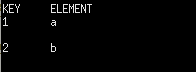## Advantage of using Map container

The main advantage of using map containers over other data structures such as arrays is if you know the keys in the map container, then you can look up the desired key in a quick time.

It avoids the fuss of iterating through the whole list as in arrays and maintains a reasonable time complexity of O(log(n)).

Further, with a huge number of elements, arrays took up a lot of space to save the memory addresses of the elements, but the map container takes only two spots to save the memory addresses.

## Functions associated with Maps

### #begin()

The begin() function returns a bidirectional iterator pointing to the very first element of the Map. This function demands no parameter to be passed and shows an error when it is done.

See the following syntax.

```mapcontainer_name.begin();
```

See the following program.

```#include <iostream>
#include <iterator>
#include <map>
using namespace std;
int main() {
map<int, char> map1;
map<int, char>::iterator cursor;
map1='a';
map1='b';
map1.begin();
}
```

### #end()

The end() function is meant to return a bidirectional iterator pointing to the element next to the last element in the map container.

Like the begin() function, this too doesn’t require any parameter. Also, since it points to a non-valid element, it cannot be dereferenced.

See the following syntax.

```mapcontainer_name.end();
```

See the following program.

```#include <iostream>
#include <iterator>
#include <map>
using namespace std;
int main() {
map<int, char> map1;
map<int, char>::iterator cursor;
map1='a';
map1='b';
map1.end();
}
```

### #size()

The size() function returns the number of elements of the map container.

The thing to note here is that the key-value pair and mapped value are considered a single element.

See the following syntax.

```mapcontainer_name.size();
```

See the following code.

```#include <iostream>
#include <iterator>
#include <map>
using namespace std;
int main() {
map<int, char> map1;
map1='a';
map1='b';
cout <<map1.size()<<endl;
}```

See the following output.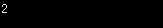### #empty()

The empty() function is a boolean function that is used to check whether the given map container is empty or not.

It returns a True value if the map container is empty, otherwise False. It takes a constant amount of time to implement this function, having the time complexity of O(1).

See the following syntax.

`mapcontainer_name.empty();`

See the following program.

```#include <iostream>
#include <iterator>
#include <map>
using namespace std;
int main() {
map<int, char> map1;
map1='a';
map1='b';
cout <<map1.empty()<<endl;
}```

See the following output.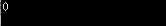### #insert()

The insert function is implemented to insert elements in the map container. It requires a key and a mapped value.

Further, it returns a bidirectional iterator pointing to the key-value inserted. See the following syntax.

```mapcontainer_name.insert({key_value, mapped_value});
```

See the following program.

```#include <iostream>
#include <iterator>
#include <map>
using namespace std;
int main()
{
map<int, char> map1;
map<int, char>::iterator cursor;
map1 = 'a';
map1 = 'b';
cout << "Original Container" << endl;
cout << "KEY\tELEMENT" << endl;
for (cursor = map1.begin(); cursor != map1.end(); cursor++)
{
cout << cursor->first;
cout << '\t' << cursor->second << '\n'
<< endl;
}
map1.insert({3, 'c'});
cout << "Updated Container" << endl;
cout << "KEY\tELEMENT" << endl;
for (cursor = map1.begin(); cursor != map1.end(); cursor++)
{
cout << cursor->first;
cout << '\t' << cursor->second << '\n'
<< endl;
}
}
```

See the following output.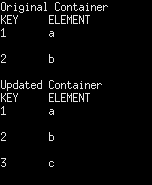### #erase()

The erase function removes a key-value pair from the map container.

It takes the key-value or the current iterator position as an argument to delete the element.

Also, it returns one(1) if the element is found and deleted, zero(0) otherwise.

See the following syntax.

```mapcontainer_name.erase(key_value);
OR
mapcontainer_name.erase(iterator_position);
```

See the following output.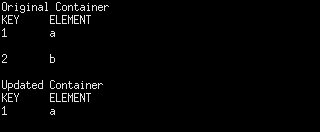### #clear()

A clear function is implemented to remove all the elements of a map container.

It takes no parameters and afterward leaves the size of the Map to be zero(0).

See the following syntax.

`mapcontainer_name.clear();`

See the following program.

```#include <iostream>
#include <iterator>
#include <map>
using namespace std;
int main()
{
map<int, char> map1;
map<int, char>::iterator cursor;
map1 = 'a';
map1 = 'b';
cout << "Original Container" << endl;
cout << "KEY\tELEMENT" << endl;
for (cursor = map1.begin(); cursor != map1.end(); cursor++)
{
cout << cursor->first;
cout << '\t' << cursor->second << '\n'
<< endl;
}
map1.clear();
cout << "Updated Container" << endl;
cout << "KEY\tELEMENT" << endl;
for (cursor = map1.begin(); cursor != map1.end(); cursor++)
{
cout << cursor->first;
cout << '\t' << cursor->second << '\n'
<< endl;
}
}
```

See the following output.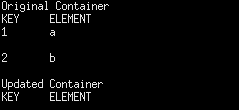### #max_size()

The max size function returns the maximum number of elements the map container can contain.

It provides the same default value, i.e., 461168601842738790, until modified.

See the following syntax.

`mapcontainer_name.max_size();`

See the following program.

```#include <iostream>
#include <iterator>
#include <map>
using namespace std;
int main()
{
map<int, char> map1;
map<int, char>::iterator cursor;
map1 = 'a';
map1 = 'b';
cout << "Original Container" << endl;
cout << "KEY\tELEMENT" << endl;
for (cursor = map1.begin(); cursor != map1.end(); cursor++)
{
cout << cursor->first;
cout << '\t' << cursor->second << '\n'
<< endl;
}
cout << "Maximum Size  " << map1.max_size() << endl;
}
```

See the following output.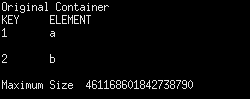## List of all the functions of Map

### #map insert()

Insert items with a particular key in the map container.

### #map count()

Returns the number of matches to items with key-value ‘g’ on the Map.

### #map equal_range()

Returns an iterator of pairs. The pair refers to the bounds of the range that includes all the items in the container, which have the key equivalent to k.

### #map erase()

Used to erase elements from the container.

### #map rend()

Returns the reverse iterator pointing to the theoretical element right before a first key-value pair in a map(considered its reverse end).

### #map rbegin()

Returns the reverse iterator, which points to the last element of the Map.

### #map find()

Returns the iterator to an item with key-value ‘g’ in the Map if found, else returns an iterator to end.

### #map crbegin()

Returns the constant reverse iterator referring to the last item in the map container.

The function crend() returns the constant reverse iterator pointing to a theoretical item before the first item in the Map.

### #map cbegin() and cend() function

Returns the constant iterator referring to the first item in the map container.

The cend() function returns the constant iterator pointing to the theoretical element that follows the last item in the multimap.

### #map emplace()

Insert the key and its item in the map container.

### #map max_size()

Returns a maximum number of items a map container can hold.

### #map upper_bound()

Return the iterator to the first item equivalent to the mapped value with a key-value ‘g’ or go after an item with key-value ‘g’ on the Map.

### #map operator

Assigns the contents of the container to a different container, replacing its current content.

### #map lower_bound()

Returns an iterator to the first item equivalent to the mapped value with a key-value ‘g’ or definitely will not go before the item with key-value ‘g’ on the Map.

### #map emplace_hint()

Insert the key and its item in the map container with a hint.

### #map value_comp()

Returns the object that determines how the items in the Map are ordered (‘<‘ by default).

### #map key_comp()

Returns the object that determines how the items in the Map are ordered (‘<‘ by default).

### #map::size()

Returns the number of items on the map.

### #map::empty()

Returns whether the map is empty.

### #map::begin() and end()

Returns an iterator to the first item in the map.

The function end() returns an iterator to the theoretical element that follows the last element in the Map.

### #map::operator[]

The operator is used to reference the element present at the position given inside the operator.

### #map::clear()

Remove all the items from the map.

### #map::at() and map::swap()

The () function returns the reference to the item associated with a key k.

The swap() function is used to exchange the contents of two maps, but the maps must be of the same type, although sizes may differ.

That’s it for this tutorial.

Categories C++

This site uses Akismet to reduce spam. Learn how your comment data is processed.